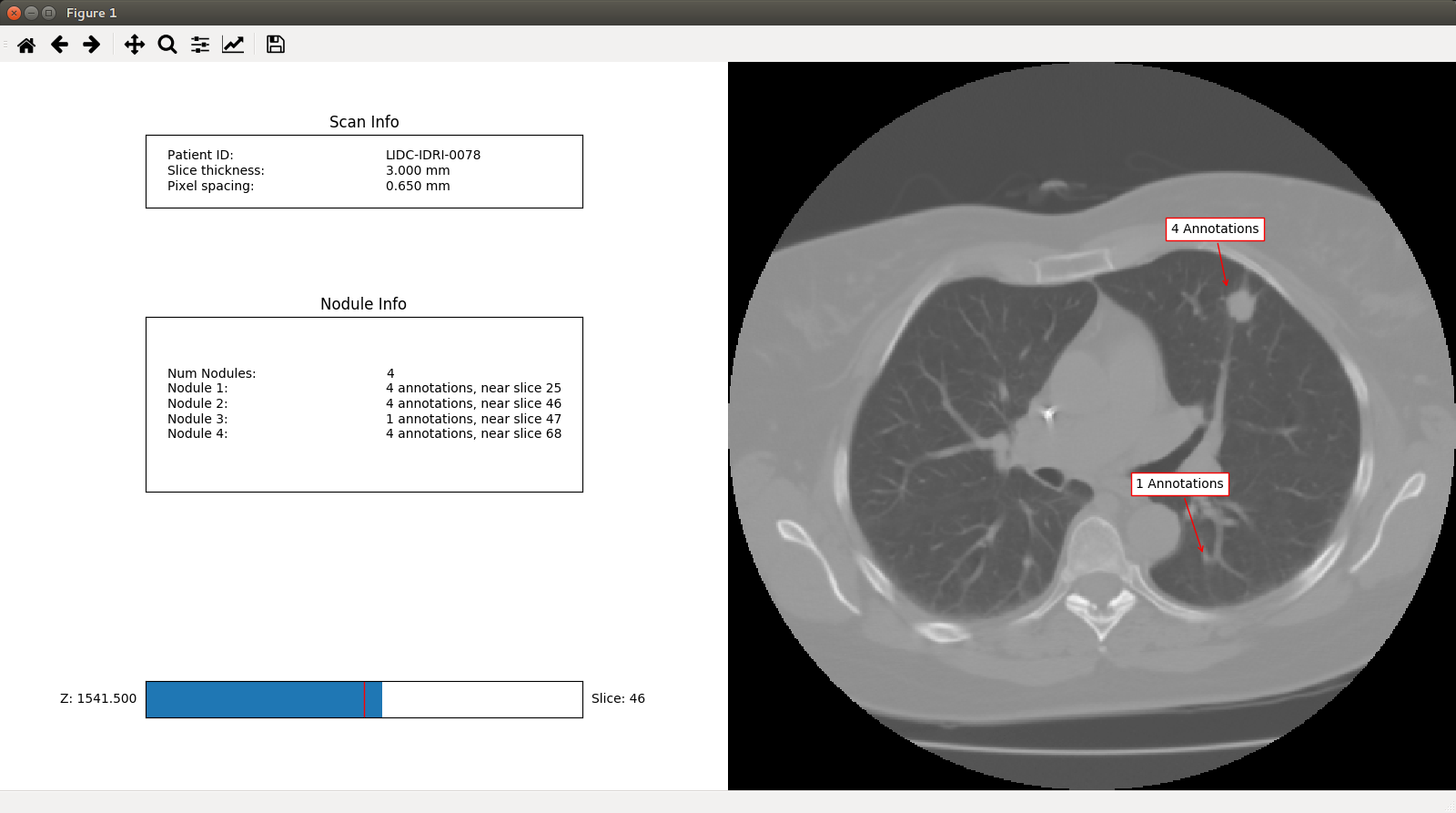# The Scan class¶

The `pylidc.Scan` class holds some (but not all!) of the DICOM attributes associated with the CT scans in the LIDC dataset. These attributes can be used to query the data:

```import pylidc as pl

# Query for all CT scans with desired traits.
scans = pl.query(pl.Scan).filter(pl.Scan.slice_thickness <= 1,
pl.Scan.pixel_spacing <= 0.6)
print(scans.count())
# => 31

pid = 'LIDC-IDRI-0078'
scan = pl.query(pl.Scan).filter(pl.Scan.patient_id == pid).first()
```

Note

Not all of the class attributes are queryable! Some attributes are actually computed properties. You can determine this by, for example, examining the object type for the class:

```print(type(pl.Scan.slice_thickness))
# => <class 'sqlalchemy.orm.attributes.InstrumentedAttribute'>
# => ^^^ queryable because it's an sqlalchemy attribute.

# Whereas ...
print(type(pl.Scan.slice_spacing))
# => <type 'property'>
# ^^^ not queryable because it's a computed property
```

The slice_thickness attribute corresponds to the DICOM attribute (0018,0050), whereas the slice_spacing attribute is computed from the median of spacing between the slices (see `pylidc.Scan.slice_zvals()`).

A `pylidc.Scan` object has zero or more `pylidc.Annotation` objects, which are radiologist annotations of lung nodules found in the scan:

```print(len(scan.annotations))
# => 13
```

## Annotation clustering¶

The scan has 13 annotations, but which refer to the same nodule? This can be determined using the `pylidc.Scan.cluster_annotations()` method, which uses a distance function to create an adjancency graph to determine which annotations refer to the same nodule in a scan:

```nods = scan.cluster_annotations()

print("%s has %d nodules." % (scan, len(nods)))
# => Scan(id=1,patient_id=LIDC-IDRI-0078) has 4 nodules.

for i,nod in enumerate(nods):
print("Nodule %d has %d annotations." % (i+1, len(nods[i])))
# => Nodule 1 has 4 annotations.
# => Nodule 2 has 4 annotations.
# => Nodule 3 has 1 annotations.
# => Nodule 4 has 4 annotations.
```

## Converting scan image values to NumPy array¶

The scan can be converted to a NumPy array for image-processing:

```vol = scan.to_volume()
print(vol.shape)
# => (512, 512, 87)

print("%.2f, %.2f" % (vol.mean(), vol.std()))
# => -702.15, 812.52
```

## Scan visualization¶

An interactive GUI can be engaged, with clustered annotations optionally indicated with arrows:

```scan.visualize(annotation_groups=nods)
```

which appears like